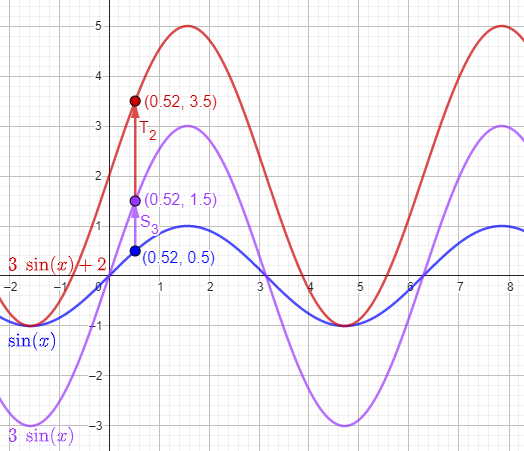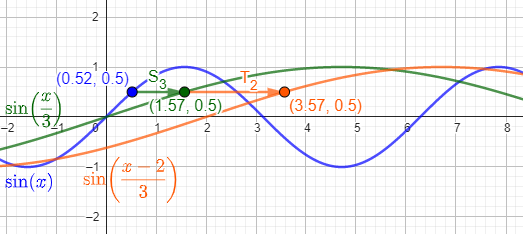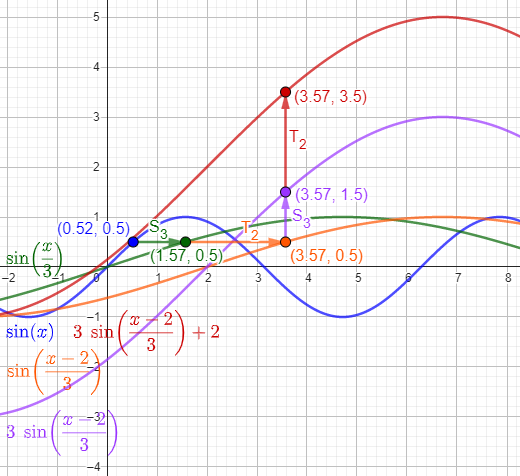# Function Transformations as Composition

#### (A new question of the week)

We have discussed transformations of functions and their graphs at length, but a recent question suggested a slightly different way to think about them.

## Are transformation and composition related?

The question came from Anindita in mid-February:

I am studying function in order to help my son who is a student of grade 11. I am referring to the book on precalculus by Stewart. I have studied about function transformation, function combination and composition of function. What I understood is that all these three methods are producing new functions.

In case of transformation for say, y = f(x) = x^2, we get a new function say g(x) = f(x) + 2 = x^2 + 2. This is called vertical translation. Here domains of f(x) and that of g(x) are same i.e., x, but ranges are different.

But if we take range of f(x) as domain of g(x) then we are compositing f(x) and g(x). In this case output of function g is termed as g(f(x)) = x^2 + 2. If my understanding is correct, then composition of two functions are giving same output as obtained by vertical translation. Is this a particular case when is same result coming? Or, there is any generalization?

In fact my question is, what is the interconnection between transformation of function and composition of function? Are they related by any means?

### Compositions, in general, are more complicated than transformations

Doctor Fenton was the first to answer:

Hi Anindita,

While in some simple cases, function composition can be related to the simple function transformations such as the one you mentioned, in general composition is much more complicated.

You miscomputed the composition g(f(x)) for f(x)=x2 and g(x)=x2+2.

g(f(x)) = (f(x))2 + 2

= (x2)2 + 2

= x4 + 2 ,

not g(f(x)) = x2 + 2 (= g(x)).  Also, the composition in the other order is quite different:

f(g(x)) = f(x2+2)

= (x2+2)2

= x4 + 2x2 + 4   .

Neither of these compositions can be described in terms of the simple transformations (vertical shifts, horizontal shifts, vertical stretching or compression, horizontal stretching or compression, and reflection).

It’s possible that Anindita had a different composition in mind. But it’s clear that the composition she stated is not the same as any of the standard transformations.

It may be easier to see the differences between the effects of simple transformations and compositions by looking at trigonometric functions such as f(x) = sin(x).  Vertical translations shift the sine wave up or down, but don’t change its periodic character, frequency, or amplitude.  A horizontal translation sin(x+c) shifts the wave to the right or left (or doesn’t change the graph if the shift is an integer multiple of the period).  A vertical stretch  A sin(x) (if A > 1) or compression (A < 1) changes the amplitude but nothing else, but a horizontal stretch sin(kx) if (k < 1) or compression (k > 1) changes the frequency, but not the amplitude.

I, too, have observed that trig functions, once you’ve learned about them, make better examples of the transformations because they don’t have the confusing properties discussed in Equivocal Function Transformations. Each transformation stands out in the graph, as further explained in Graphing Transformed Sines.

However, if you compose h(x) = sin(x) with f(x) = x2, the resulting graph of h(f(x)) = sin(x2) is not periodic and does not have a constant frequency or amplitude, but f(h(x)) = (sin(x))2 is equal (by a trigonometric identity) to (1/2) – (1/2)cos(2x), which can be obtained from h(x) by simple transformations.

So, composition is a much more complicated type of transformation than the simple transformations.

Compositions in general are far more complicated, and can’t be described as transformations; but what about the reverse?

### Transformations can be seen as compositions

I had been pondering this from a different perspective, and joined in:

Hi, Anindita.

I have some different thoughts about this. Although not all compositions of functions are as simple as the transformations you have learned about, those transformations can be thought of as compositions of functions, and thinking about them that way can be very helpful.

For example, your vertical translation can be thought of as a function T(x) = x + 2 that adds 2 to its input, and the shifted function g(x) = x^2 + 2 is then

g(x) = T(f(x)) = T(x^2) = x^2 + 2

Here we are applying the transformation T to the value of y = f(x).

A vertical transformation applies a simple function like my T to the output of the original function: $$g=T\circ f$$.

And if you do two transformations, a vertical stretch by a factor of 3, followed by a vertical translation up 2 units, can be seen as a composition of three functions:

f(x) = x^2

S(x) = 3x

T(x) = x + 2

g(x) = T(S(f(x))) = T(S(x^2)) = T(3x^2) = 3x^2 + 2

Here, $$g=T\circ S\circ f$$.

Similarly, a horizontal shift right 2 units can be thought of as

h(x) = f(T^-1(x)) = f(x – 2) = (x – 2)^2

I discussed this idea in passing near the bottom of this page:

Function Transformations Revisited (II)

There are several more things we could discuss, if this is the sort of thing you had in mind. (Or even if it wasn’t …)

A horizontal transformation applies the inverse of a simple function like my T to the input of the original function: $$g=f\circ T^{-1}$$. We’ll see more about this below.

The page I referred to uses the concept of composition without explicitly calling it that, except when I brought in the idea of inverses. What I’m discussing here is intended to fill in that gap.

## Restating the concepts

Anindita responded to each of us, reflecting what we said:

I am studying functions first time, so I have committed errors in computations. But I understood the correction Dr Fenton did.

Referring to the reply Dr Peterson made I want to say that, this is what I wanted to understand i.e., if there is any underlying general connection between transformation and composition. Now I see that there are cases where transformations can be thought of as composition but there is no such generalization.

What comes in my mind is that, transformation is changing of domain of a function or changing the rule of function. Consider a function y = f(x) = x^2 where domain is values of x and range becomes values of x^2. If we take new domain for same function y1 = f(x-1) = (x-1)^2 then domain becomes (x-1) and range becomes (x-1)^2. Here graph of function f(x-a) seen to be shifted towards right w.r.t that of y = f(x) = x^2.

Again if we consider another function y2 = g(x) = f(x) – 1 = x^2 – 1. In this case we have taken same values of x but rules of function is changed and hence range is also changed. This causes vertical translation of  y = f(x) = x^2. So any translation is actually change in domain for same function or change in rule for a new function keeping domain same.

But composition of f(x) and g(x) is altogether different thing. Because g(f(x)) = ((x^2)^2) – 1 = x^4 – 1. Here range of f(x) becomes domain of g(x) and accordingly range of g(f(x)) varies.

Now many transformations can be represented by composition by using suitable intermediate functions. In the example y = f(x) = x^2 and g(x) = f(x) + 2 = x^2 + 2, we brought a new function T(x) = x+2 and then T(f(x)) gives same output that g(x) gives. Thus composition of f(x) and T(x) causes transformation of f(x), same as done by g(x).

Some of the terminology, that of domain and range, is wrong, but the main ideas are right.

### Clarifying what we mean by transformations

She is restating what I said, mostly correctly, but with some possible misuses of terminology, so I made a couple clarifications.

I think when you say, “transformation is changing of domain of a function or changing the rule of function”, you are perhaps using the word “transformation” more broadly than we intend. The “simple transformations” we have referred to are just the types your son is learning: translation, reflection, stretching. These are discussed in articles like this in our blog:

Shifting and Stretching Graphs

and others with links at the bottom.

There are also other transformations of graphs that one could consider, such as rotation; but that typically does not result in a function, so we restrict our attention to the three listed.

In the broad sense, a transformation can be thought of as any function that turns one function into another. Let’s stick with those your son is learning about. These transform one function to another specifically by composing a given function with one of the three basic functions,

Translation (or shift): Tk(x) = x + k

Stretch (or dilation): Sk(x) = kx

Reflection: R(x) = -x

These may change the domain or the range (according to the order in which they are applied) in simple ways, but that is secondary to their main effect, which is to modify the graph in an easily understood way.

These three functions respectively add, multiply, and negate a variable; these result in the indicated changes to a graph.

Again, although not all compositions can be described as transformations, all of the transformations we talk about can be expressed as compositions (with those three types of function I listed).

Your last paragraph is correct. We are transforming the given function f into a new function g by composition with function T, which produces a translation.

There is more to say, if this hasn’t fully answered your question. Looking at transformations as compositions leads to some important ideas.

## Transformation as composition

I understood that all transformation can be represented as composition but the reverse is not true. You gave examples also. But if possible then provide me some insight about the details of those ideas that comes while dealing with transformation of function as composition.

### Vertical translation, stretching, and reflection

I replied:

The ideas I have in mind were suggested by a recent discussion with a colleague. It may well be above your current level of understanding, but it may be of interest.

Here is an example involving vertical transformations:

To stretch a function f vertically by a factor of a, and then translate it up a distance of b, we first multiply y by a, and then add b to it. So we first evaluate y = f(x), to obtain the original value of y, then apply Sa(y) = ay, and then apply Tb(y) = y + b:

g(x) = Tb(Sa(f(x))) = Tb(af(x)) = af(x)) + b

That expresses the combination of transformations as a composition. of stretching (and/or reflection, for negative a) and translation.

To make this more concrete, let’s take a specific example. Suppose we want to take the function $$f(x)=\sin(x)$$ and first stretch it vertically by a factor of 3, then translate it upward by a distance of 2.

The stretch is accomplished by $$S_3(y) = 3y$$, multiplying the y-coordinate of each point by 3; and the translation is accomplished by $$T_2(y)=y+2$$, adding 2 to the y-coordinate. Applying these, in order, to $$f(x)$$, we get $$g(x)=T_2(S_3(f(x)))=T_2(3f(x))=3f(x))+2=3\sin(x)+2 .$$ So the new function is $$g=T_2\circ S_3\circ f .$$Here the blue curve is the original, stretched to the purple curve, then translated to the red curve, with a typical point on each shown.

If, instead, we wanted to do the translation first, we would have $$h(x)=S_3(T_2(f(x)))=S_3(f(x)+2)=3(f(x))+2)=3f(x)+6=3\sin(x)+6 .$$ So this function is $$h=S_3\circ T_2\circ f .$$ It is different because we stretched the result of the translation, multiplying the distance moved by 3.

As an aside, we can solve this to find f(x) in terms of g(x), by first subtracting b and then dividing by a:

f(x) = (g(x) – b)/a

That is, the same pair of transformations can be obtained by replacing y in the equation y = f(x) with (y – b)/a:

(y – b)/a = f(x)

If you have seen inverse functions enough, what I just did amounts to applying both inverse functions, in reverse order:

Sa-1(Tb-1(g(x))) = f(x)

(I’m ignoring reflection, which just means making “a” negative.)

The idea here is that the inverse of a composite function $$f\circ g$$ is $$g^{-1}\circ f^{-1}$$, because $$(g^{-1}\circ f^{-1})\circ(f\circ g)=(g^{-1}\circ (f^{-1})\circ))f\circ g=(g^{-1}\circ Id)\circ g=g^{-1}\circ g=Id .$$

In our example, $$g(x)=3\sin(x)+2$$, so $$\sin(x)=\frac{g(x)-2}{3}=\frac{T_{-2}(g(x))}{3}=S_{\frac{1}{3}}(T_{-2}(g(x))) .$$

We’ll use this idea in a moment.

### Horizontal translation, stretching, and reflection

Now, if we want to stretch our function horizontally by a factor of c, and then translate it horizontally by a distance of d, the new point at x on the graph of g has to be the result of stretching and shifting from some x’ on the graph of f; so the input to f has to be a value x’ such that Td(Sc(x’))) = x. To find x’, we solve that equation, which is

cx’ + d = x ,

whose solution is

x’ = (x – d)/c .

In terms of the functions,

x’ = Sc-1(Td-1(x)).

So we want to use f(x’) = f( Sc-1(Td-1(x))).

To be specific, suppose we want to take the function $$f(x)=\sin(x)$$ and first stretch it horizontally by a factor of 3, then translate it right by a distance of 2. Then a given point $$(k,\sin(k))$$ on the original graph will move to a point $$(3k+2,\sin(k))$$ on the new graph, because x has to be doubled, then increased by 2, but y stays the same. Therefore, the new function g has to satisfy the equation $$g(3k+2)=\sin(k) .$$

In terms of composition, $$g\circ T_2\circ S_3=\sin,$$ and solving this, we get $$g=\sin\circ S_3^{-1}\circ T_2^{-1}=\sin\circ S_{\frac{1}{3}}\circ T_{-2}\\g(x)=\sin\left(\frac{x-2}{3}\right) .$$Here the blue curve is the original, stretched horizontally to the green curve, then translated to the orange curve.

### Combining it all

The result of doing all four transformations is therefore

g(x) = Tb(Sa(f(Sc-1(Td-1(x)))))

= Tb(Sa(f(Sc-1(x – d))))

= Tb(Sa(f((x – d)/c)))

= Tb(af((x – d)/c))

= Tb(af((x – d)/c))

af((x – d)/c)) + b .

That is the transformed function as shown in

Combining Function Transformations: Order Matters

For our example, this is $$h=T_2\circ S_3\circ \sin\circ S_3^{-1}\circ T_2^{-1}\\h(x)=3\sin\left(\frac{x-2}{3}\right)+2 .$$Here we have continued from the previous graph, stretching the orange curve vertically to the purple curve, then translating vertically to the red curve.

Notice that we replace x with Sc-1(Td-1(x)) = (x – d)/c, just as we earlier saw that we could replace y with (y – b)/a. So in this form, the transformed equation looks like

(y – b)/a = f((x – d)/c)

This is the form my colleague, a retired professor, came up with when faced with this sort of problem in a precalculus setting unfamiliar to him.

Anindita replied, with a comment we commonly see about schools in certain countries:

You are helping us to learn this subject in a totally different way which we did not learn in our school days. Even today schools teach us just methods, but intuitive feelings and how or why these methods work are not generally discussed in schools. Seeing any topic from different perspectives helps to grasp the deeper understanding. You are giving us that opportunity. I am truly grateful to you for your effort. Without depending on just schools or private tutors who just teach methods and depend on rote learning I teach him myself by studying books and following the path that you show me. Moreover from your busy schedule you are taking time for us. So I am and will always remain grateful to you.

Regarding your suggestion about dealing with transformation of function as composition, this is really a very interesting idea. I am thinking about it. I need time and practice to fully grasp the idea, and I will share with my son the gist of it that how combination of transformation can be expressed as composition. If any question comes in my mind then I will ask. Thanks a lot again for your patience.

I expected this to require time to digest, as I really wrote it to satisfy my desire to write out these ideas, which I have only hinted at before. Anindita seems like the kind of person who is up to the challenge!

The conversation continued with further questions about functions, including the one that led me to write the last two posts.

This site uses Akismet to reduce spam. Learn how your comment data is processed.